# DAV Class 4 Maths Chapter 2 Worksheet 5 Solutions

The DAV Class 4 Maths Book Solutions and DAV Class 4 Maths Chapter 2 Worksheet 5 Solutions of Addition and Subtraction offer comprehensive answers to textbook questions.

## DAV Class 4 Maths Ch 2 WS 5 Solutions

Question 1.
Subtract:
(a) 78,321 from 85,213
Arrange in column. Then solve each question.(b) 96,570 from 97,295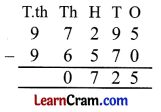(c) 62,783 from 71,057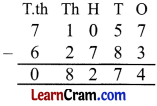(d) 88,782 from 89,560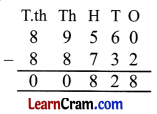(e) 42,150 from 50,000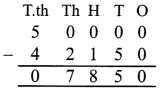(f) 53,008 from 79,096Question 2.
Find the difference between:
(a) 6,54,372 and 1,28,453
6,54,372 and 1,28,453
Comparing lakh place we get
6 > 1
∴ 6,54,372 > 1,28,453
Now arrange in column.(b) 9,76,517 and 5,28,753
As 9 > 5
∴ 9,76,517 and 5,28,753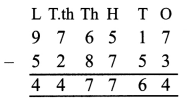(c) 5,484 and 7,98,454
As 5,484 and 7,98,454
First we count number of digits.
As 7,98,454 has more digits than 5,484 so.
7,98,454 > 5,484
Now arrange in column.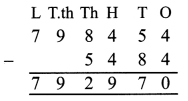(d) 57,357 and 2,99,059
First count the digits.
As 2,99,059 has more digits than 57,357
2,99,059 > 57,357
Now arrange in column.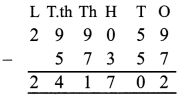(e) 9,76,287 and 62,578
9,76,287 and 62,578 as 9,76,287
Arrange in column.(f) 64,686 and 9,86,468
64,686 and 9,86,468 as 64,686 < 9,86,468
so we subtract 9,86,468 to 64,686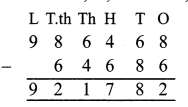Question 3.
Perform the following subtraction and check the answer.
(a) 9,347 – 1,251
To check: sum of subtrahend and difference should be equal to minuend,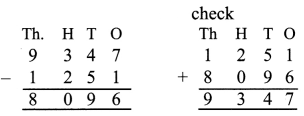(b) 6,792 – 4,321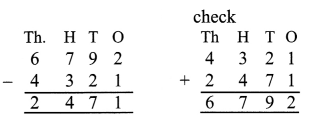(c) 62,546 – 61,777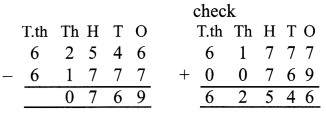(d) 1,90,000 – 89,999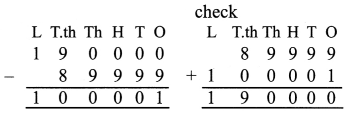(e) 6,98,527 – 2,56,798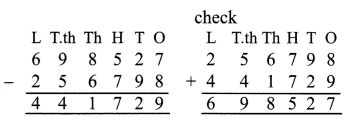(f) 5,43,922 – 4,31,789Question 4.
Find the difference between the largest number of five digits and the smallest number of three digits.
Largest number of 5-digits = 99999
Smallest number of 3-digits = 100
difference = 99899### DAV Class 4 Maths Chapter 2 Worksheet 5 Notes

Subtraction (5-Digit Number And 6-Digit Number)E.g. 1.
Subtract 42565 from 80596Explanation:
(a) Subtracting ones:
6 ones – 5 ones = 1 ones.
(b) Subtracting tens:
9 tens – 6 tens = 3 tens.
(c) Subtracting hundreds:
5 hundreds – 5 hundreds = 0 hundreds.
(d) Subtracting thousands:
We cannot subtract 0. So, we borrow 1 ten thousands from ten thousands column. Now subtract
10 thousands – 2 thousands = 8 thousands
(e) Subtracting ten thousands:
7 ten thousands – 4 ten thousands
= 3 ten thousands

E.g. 2.
Subtract 3,30,256 from 7,98,450.